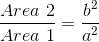ISEE Middle Level Math : Quadrilaterals

Example Questions

Example Question #21 : Squares

Find the perimeter of a square with a width of 9in.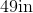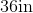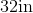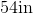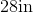Explanation:

To find the perimeter of a square, we will use the following formula: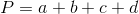where a, b, c, and d are the lengths of the sides of the square.

Now, we know the width of the square is 9in.  Because it is a square, all sides are equal. Therefore, all sides are 9in.  So, we can substitute.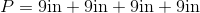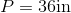Example Question #22 : Squares

Find the perimeter of a square with a width of 9cm.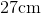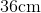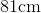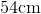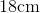Explanation:

To find the perimeter of a square, we will use the following formula:where a, b, c, and d are the lengths of the sides of the square.

Now, we know the width of the square is 9cm.  Because it is a square, all sides are equal.  Therefore, all sides are 9cm.  So, we can substitute.  We get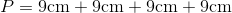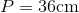Example Question #23 : Squares

Find the perimeter of a square with a length of 6in.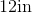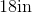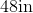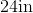Explanation:

To find the perimeter of a square, we will use the following formula:where a, b, c, and d are the lengths of the sides of the square.

Now, we know the length of the square is 6in.  Because it is a square, all sides are equal.  Therefore, all sides are 6in.  So, we can substitute.  We get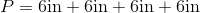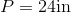Example Question #24 : Squares

Find the perimeter of a square with a length of 14cm.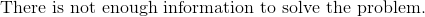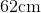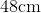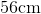Explanation:

To find the perimeter of a square, we will use the following formula:where a, b, c, and d are the lengths of the sides of the square.

Now, we know the width of the square is 14cm.  Because it is a square, all sides are equal.  Therefore, all sides are 14cm.  So, we get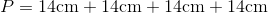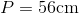Example Question #25 : Squares

Find the perimeter of a square that has a length of 13in.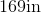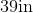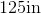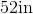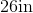Explanation:

To find the perimeter of a square, we will use the following formula:where a, b, c, and d are the lengths of the sides of the square.

Now, we know the length of the square is 13in.  Because it is a square, all sides are equal.  Therefore, all sides are 13in.

Knowing this, we can substitute into the formula.  We get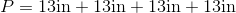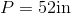Example Question #26 : Squares

Which of the following is equal to the area of a square with perimeter 8 meters?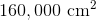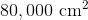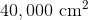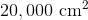Explanation:

The sidelength of a square is one-fourth its perimeter, so the sidelength here is one-fourth of 8, or 2, meters. One meter is equal to 100 centimeters, so the sidelength is 200 centimeters. Square this to get the area: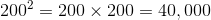square centimeters.

Example Question #27 : Squares

Which of the following is the area of a square with perimeter 7 feet?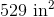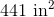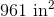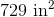Explanation:

Convert  7 feet to inches by multiplying by 12: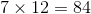inches.

The sidelength of a square is one-fourth its perimeter, so the sidelength here is one-fourth of 84 inches. This is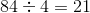inches.

Square this to get the area: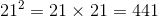square inches

Example Question #1 : How To Find The Area Of A Square

What is the area of a square with a side length of 4?

12

16

14

4

8

16

Explanation:

The area of a square is represented by the equation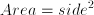.

Therefore the area of this square is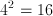.

Example Question #2 : How To Find The Area Of A Square

Square A has sides measuring 5 meters.  A second square, Square B, has sides that are 2 meters longer than the sides of Square A.  What is the difference in area of Square A and Square B?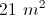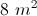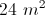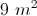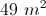Explanation:

The area of Square A is 5 * 5, or 25 m2.

Since each of Square B's sides is 2 meters longer, the sides measure 7 meters. Therefore, the area of square B is 49 m2.

Subtract to find the difference in areas: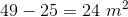Example Question #30 : Squares

The ratio of the length of a side of one square to the length of the side of another square is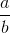. Give the ratio of the area of the second square to the area of the first square.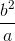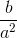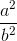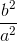Explanation:

The area of a square can be found as follows: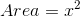Where: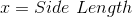So we can write: